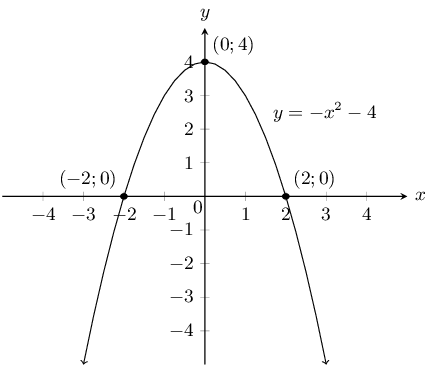Home Practice
For learners and parents For teachers and schools
Textbooks
Full catalogue
Pricing SupportLog in

We think you are located in United States. Is this correct?

### Functions of the form $$y={x}^{2}$$ (EMA4J)

Functions of the general form $$y=a{x}^{2}+q$$ are called parabolic functions. In the equation $$y=a{x}^{2}+q$$, $$a$$ and $$q$$ are constants and have different effects on the parabola.

## Worked example 4: Plotting a quadratic function

$$y=f(x)={x}^{2}$$

Complete the following table for $$f(x)={x}^{2}$$ and plot the points on a system of axes.

 $$x$$ $$-\text{3}$$ $$-\text{2}$$ $$-\text{1}$$ $$\text{0}$$ $$\text{1}$$ $$\text{2}$$ $$\text{3}$$ $$f(x)$$ $$\text{9}$$
1. Join the points with a smooth curve.

2. The domain of $$f$$ is $$x\in \mathbb{R}$$. Determine the range.

3. About which line is $$f$$ symmetrical?

4. Determine the value of $$x$$ for which $$f(x)=6\frac{1}{4}$$. Confirm your answer graphically.

5. Where does the graph cut the axes?

### Substitute values into the equation

\begin{align*} f(x) & = {x}^{2} \\ f(-3) & = {(-3)}^{2} = 9\\ f(-2) & = {(-2)}^{2} = 4\\ f(-1) & = {(-1)}^{2} = 1\\ f(0) & = {(0)}^{2} = 0\\ f(1) & = {(1)}^{2} = 1\\ f(2) & = {(2)}^{2} = 4\\ f(3) & = {(3)}^{2} = 9 \end{align*}
 $$x$$ $$-\text{3}$$ $$-\text{2}$$ $$-\text{1}$$ $$\text{0}$$ $$\text{1}$$ $$\text{2}$$ $$\text{3}$$ $$f(x)$$ $$\text{9}$$ $$\text{4}$$ $$\text{1}$$ $$\text{0}$$ $$\text{0}$$ $$\text{4}$$ $$\text{9}$$

### Plot the points and join with a smooth curve

From the table, we get the following points:

$$(-3;9), (-2;4), (-1;1), (0;0), (1;1), (2;4), (3;9)$$

### Determine the domain and range

Domain: $$x\in \mathbb{R}$$

From the graph we see that for all values of $$x$$, $$y \ge 0$$.

Range: $$\left\{y:y\in \mathbb{R}, y\ge 0\right\}$$

### Find the axis of symmetry

$$f$$ is symmetrical about the $$y$$-axis. Therefore the axis of symmetry of $$f$$ is the line $$x=0$$.

### Determine the $$x$$-value for which $$f(x)=6\frac{1}{4}$$

\begin{align*} f(x)& = \frac{25}{4} \\ \therefore \frac{25}{4}& = {x}^{2} \\ x& = ±\frac{5}{2}\\ & = ±2\frac{1}{2} \end{align*}

See points $$A$$ and $$B$$ on the graph.

### Determine the intercept

The function $$f$$ intercepts the axes at the origin $$(0;0)$$.

We notice that as the value of $$x$$ increases from $$-\infty$$ to $$\text{0}$$, $$f(x)$$ decreases.

At the turning point $$(0;0)$$, $$f(x)=0$$.

As the value of $$x$$ increases from $$\text{0}$$ to $$∞$$, $$f(x)$$ increases.

## The effects of $$a$$and $$q$$ on a parabola.

Complete the table and plot the following graphs on the same system of axes:

1. $${y}_{1}={x}^{2}-2$$

2. $${y}_{2}={x}^{2}-1$$

3. $${y}_{3}={x}^{2}$$

4. $${y}_{4}={x}^{2}+1$$

5. $${y}_{5}={x}^{2}+2$$

 $$x$$ $$-\text{2}$$ $$-\text{1}$$ $$\text{0}$$ $$\text{1}$$ $$\text{2}$$ $${y}_{1}$$ $${y}_{2}$$ $${y}_{3}$$ $${y}_{4}$$ $${y}_{5}$$

Use your results to deduce the effect of $$q$$.

Complete the table and plot the following graphs on the same system of axes:

1. $${y}_{6}=-2{x}^{2}$$

2. $${y}_{7}=-{x}^{2}$$

3. $${y}_{8}={x}^{2}$$

4. $${y}_{9}=2{x}^{2}$$

 $$x$$ $$-\text{2}$$ $$-\text{1}$$ $$\text{0}$$ $$\text{1}$$ $$\text{2}$$ $${y}_{6}$$ $${y}_{7}$$ $${y}_{8}$$ $${y}_{9}$$

Use your results to deduce the effect of $$a$$.

The effect of $$q$$

The effect of $$q$$ is called a vertical shift because all points are moved the same distance in the same direction (it slides the entire graph up or down).

• For $$q>0$$, the graph of $$f(x)$$ is shifted vertically upwards by $$q$$ units. The turning point of $$f(x)$$ is above the $$y$$-axis.

• For $$q<0$$, the graph of $$f(x)$$ is shifted vertically downwards by $$q$$ units. The turning point of $$f(x)$$ is below the $$y$$-axis.

The effect of $$a$$

The sign of $$a$$ determines the shape of the graph.

• For $$a>0$$, the graph of $$f(x)$$ is a “smile” and has a minimum turning point at $$(0;q)$$. The graph of $$f(x)$$ is stretched vertically upwards; as $$a$$ gets larger, the graph gets narrower.

For $$0<a<1$$, as $$a$$ gets closer to $$\text{0}$$, the graph of $$f(x)$$ gets wider.

• For $$a<0$$, the graph of $$f(x)$$ is a “frown” and has a maximum turning point at $$(0;q)$$. The graph of $$f(x)$$ is stretched vertically downwards; as $$a$$ gets smaller, the graph gets narrower.

For $$-1<a<0$$, as $$a$$ gets closer to $$\text{0}$$, the graph of $$f(x)$$ gets wider.

 $$a<0$$ $$a>0$$ $$q>0$$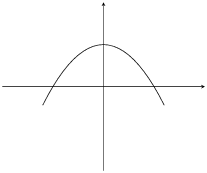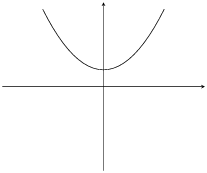$$q=0$$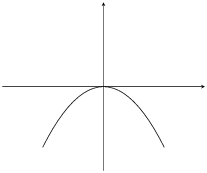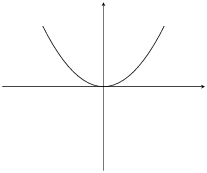$$q<0$$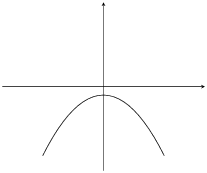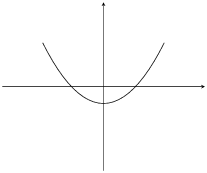Table 6.2: The effect of $$a$$ and $$q$$ on a parabola.

You can use this Phet simulation to help you see the effects of changing $$a$$ and $$q$$ for a parabola.

### Discovering the characteristics (EMA4M)

The standard form of the equation of a parabola is $$y=a{x}^{2}+q$$.

#### Domain and range

The domain is $$\left\{x:x\in \mathbb{R}\right\}$$ because there is no value for which $$f(x)$$ is undefined.

If $$a>0$$ then we have:

$\begin{array}{cccl} {x}^{2}& \ge & 0 & (\text{Perfect square is always positive}) \\ a{x}^{2}& \ge & 0 & (\text{since } a>0) \\ a{x}^{2}+q & \ge & q & (\text{add } q \text{ to both sides}) \\ \therefore f(x) & \ge & q & \end{array}$

Therefore if $$a>0$$, the range is $$\left[q;\infty \right)$$. Similarly, if $$a<0$$ then the range is $$\left(-\infty ;q\right]$$.

## Worked example 5: Domain and range of a parabola

If $$g(x)={x}^{2}+2$$, determine the domain and range of the function.

### Determine the domain

The domain is $$\left\{x:x\in \mathbb{R}\right\}$$ because there is no value for which $$g(x)$$ is undefined.

### Determine the range

The range of $$g(x)$$ can be calculated as follows:

\begin{align*} {x}^{2}& \ge 0 \\ {x}^{2}+2& \ge 2\\ g(x)& \ge 2 \end{align*}

Therefore the range is $$\left\{g\left(x\right):g\left(x\right)\ge 2\right\}$$.

#### Intercepts

The $$y$$-intercept:

Every point on the $$y$$-axis has an $$x$$-coordinate of $$\text{0}$$, therefore to calculate the $$y$$-intercept let $$x=0$$.

For example, the $$y$$-intercept of $$g(x)={x}^{2}+2$$ is given by setting $$x=0$$:

\begin{align*} g(x)& = {x}^{2}+2 \\ g(0)& ={0}^{2}+2\\ & =2 \end{align*}

This gives the point $$(0;2)$$.

The $$x$$-intercepts:

Every point on the $$x$$-axis has a $$y$$-coordinate of $$\text{0}$$, therefore to calculate the $$x$$-intercept let $$y=0$$.

For example, the $$x$$-intercepts of $$g(x)={x}^{2}+2$$ are given by setting $$y=0$$:

\begin{align*} g(x)& = {x}^{2}+2 \\ 0 & = {x}^{2}+2 \\ -2& = {x}^{2} \end{align*}

There is no real solution, therefore the graph of $$g(x)={x}^{2}+2$$ does not have $$x$$-intercepts.

#### Turning points

The turning point of the function of the form $$f(x)=a{x}^{2}+q$$ is determined by examining the range of the function.

• If $$a>0$$, the graph of $$f(x)$$ is a “smile” and has a minimum turning point at $$(0;q)$$.

• If $$a<0$$, the graph of $$f(x)$$ is a “frown” and has a maximum turning point at $$(0;q)$$.

#### Axes of symmetry

The axis of symmetry for functions of the form $$f(x)=a{x}^{2}+q$$ is the $$y$$-axis, which is the line $$x=0$$.

### Sketching graphs of the form $$y=a{x}^{2}+q$$ (EMA4N)

In order to sketch graphs of the form $$f(x)=a{x}^{2}+q$$, we need to determine the following characteristics:

1. sign of $$a$$

2. $$y$$-intercept

3. $$x$$-intercept

4. turning point

## Worked example 6: Sketching a parabola

Sketch the graph of $$y={2x}^{2}-4$$. Mark the intercepts and turning point.

### Examine the standard form of the equation

We notice that $$a>0$$. Therefore the graph is a “smile” and has a minimum turning point.

### Calculate the intercepts

For the $$y$$-intercept, let $$x=0$$:

\begin{align*} y& = 2{x}^{2}-4 \\ & = 2{(0)}^{2}-4 \\ & =-4 \end{align*}

This gives the point $$(0;-4)$$.

For the $$x$$-intercepts, let $$y=0$$:

\begin{align*} y& = 2{x}^{2}-4 \\ 0& = 2{x}^{2}-4 \\ {x}^{2}& = 2 \\ \therefore x& = ±\sqrt{2} \end{align*}

This gives the points $$(-\sqrt{2};0)$$ and $$(\sqrt{2};0)$$.

### Determine the turning point

From the standard form of the equation we see that the turning point is $$(0;-4)$$.

### Plot the points and sketch the graph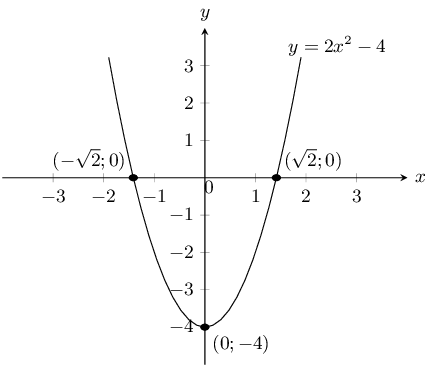Domain: $$\left\{x:x\in \mathbb{R}\right\}$$

Range: $$\left\{y:y\ge -4,y\in \mathbb{R}\right\}$$

The axis of symmetry is the line $$x=0$$.

## Worked example 7: Sketching a parabola

Sketch the graph of $$g(x)=-\frac{1}{2}{x}^{2}-3$$. Mark the intercepts and the turning point.

### Examine the standard form of the equation

We notice that $$a<0$$. Therefore the graph is a “frown” and has a maximum turning point.

### Calculate the intercepts

For the $$y$$-intercept, let $$x=0$$:

\begin{align*} g(x)& = -\frac{1}{2}{x}^{2}-3 \\ g(0)& = -\frac{1}{2}{(0)}^{2}-3 \\ & = -3 \end{align*}

This gives the point $$(0;-3)$$.

For the $$x$$-intercepts let $$y=0$$:

\begin{align*} 0& = -\frac{1}{2}{x}^{2}-3 \\ 3& = -\frac{1}{2}{x}^{2} \\ -2(3)& = {x}^{2} \\ -6& = {x}^{2} \end{align*}

There is no real solution, therefore there are no $$x$$-intercepts.

### Determine the turning point

From the standard form of the equation we see that the turning point is $$(0;-3)$$.

### Plot the points and sketch the graph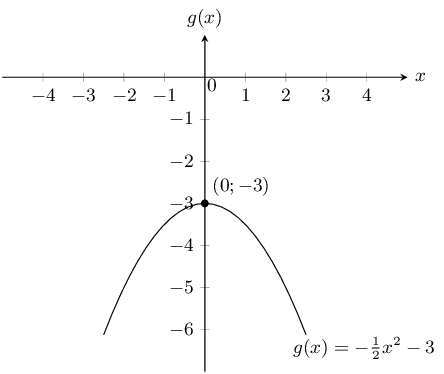Domain: $$x\in \mathbb{R}$$.

Range: $$y\in \left(-\infty ;-3\right]$$.

The axis of symmetry is the line $$x=0$$.

Textbook Exercise 6.3

The graph below shows a quadratic function with the following form: $$y = ax^2 + q$$. Two points on the parabola are shown: Point A, the turning point of the parabola, at $$(0;4)$$, and Point B is at $$\left(2; \frac{8}{3}\right)$$. Calculate the values of $$a$$ and $$q$$.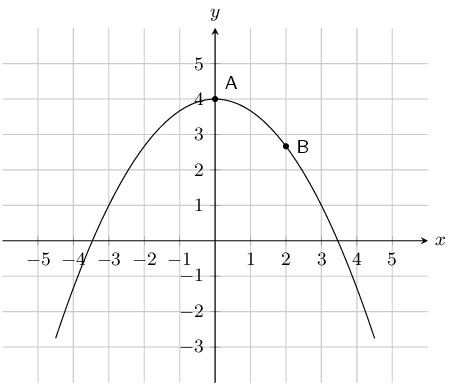The value of $$q$$ is 4.

\begin{align*} y & = ax^2 +4 \\ \left( \frac{8}{3} \right) & = a(\text{2})^2 +4 \quad \longleftarrow \quad ^{\text{substitute in the}}_{\text{coordinates of a point!}} \\ \frac{8}{3} & = \text{4}a +4 \\ \frac{8}{3} -4 & = \text{4}a \\ - \frac{4}{3} & = \text{4}a \\ - \frac{1}{3} & = a \end{align*} $a = - \frac{1}{3};~ q = 4$

The graph below shows a quadratic function with the following form: $$y = ax^2 + q$$. Two points on the parabola are shown: Point A, the turning point of the parabola, at $$(0;-3)$$, and Point B is at $$\left(2; 5\right)$$. Calculate the values of $$a$$ and $$q$$.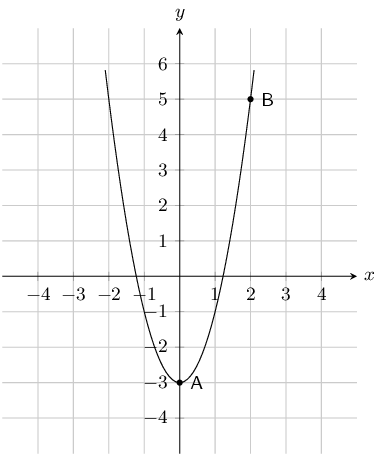The value of $$q$$ is -3.

\begin{align*} y & = ax^2 -3 \\ \left( 5 \right) & = a(\text{2})^2 -3 \quad \longleftarrow \quad ^{\text{substitute in the}}_{\text{coordinates of a point!}} \\ 5 & = \text{4}a -3 \\ 5 +3 & = \text{4}a \\ 8 & = \text{4}a \\ 2 & = a \end{align*} $a = 2;~ q = -3$

Given the following equation:

$$y=5 x^{2} - 2$$

Calculate the $$y$$-coordinate of the $$y$$-intercept.

\begin{align*} y & = ax^2 + q \\ & = 5 x^{2} - 2 \\ & = 5 (0)^{2} - 2\\ & = 0-2 \end{align*}

The $$y$$-coordinate of the $$y$$-intercept is $$-2$$.

Now calculate the $$x$$-intercepts. Your answer must be correct to 2 decimal places.

\begin{align*} y & = 5 x^{2} - 2 \\ (0) & =5 x^{2} - 2 \\ - 5 x^{2} &=-2\\ x^2 &= \frac{-2}{-5} \\ x & =\pm \sqrt{\frac{2}{5}}\\ \text{Therefore: } x = +\sqrt{\frac{2}{5}} &\text{ and } x = - \sqrt{\frac{2}{5}} \\ x= -\text{0,63} &\text{ and } x= \text{0,63} \end{align*}

The $$x$$-intercepts are $$(-\text{0,63};0)$$ and $$(\text{0,63};0)$$.

Given the following equation:

$$y=- 2 x^{2} + 1$$

Calculate the $$y$$-coordinate of the $$y$$-intercept.

\begin{align*} y & = ax^2 + q \\ & = - 2 x^{2} + 1 \\ & = - 2 (0)^{2} + 1\\ & = 0 + 1 \end{align*}

The $$y$$-coordinate of the $$y$$-intercept is $$\text{1}$$.

Now calculate the $$x$$-intercepts. Your answer must be correct to 2 decimal places.

\begin{align*} y & = - 2 x^{2} + 1 \\ (0) & =- 2 x^{2} + 1 \\ 2 x^{2} &=1\\ x^2 &= \frac{1}{2} \\ x & =\pm \sqrt{\frac{1}{2}}\\ \text{Therefore: } x = +\sqrt{\frac{1}{2}} &\text{ and } x = - \sqrt{\frac{1}{2}} \\ x= -\text{0,71} & \text{ and } x\end{align*}

The $$x$$-intercepts are $$(-\text{0,71};0)$$ and $$(\text{0,71};0)$$.

Given the following graph, identify a function that matches each of the following equations: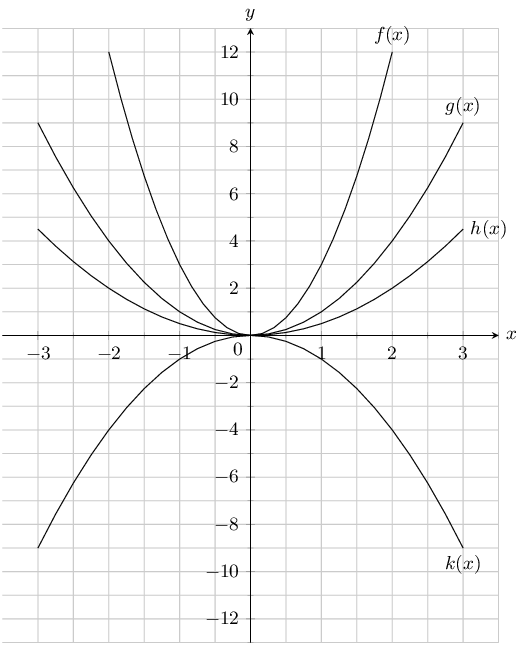$$y = \text{0,5}x^2$$

$$h(x)$$

$$y = x^2$$

$$g(x)$$

$$y = 3x^2$$

$$f(x)$$

$$y = -x^2$$

$$k(x)$$

Given the following graph, identify a function that matches each of the following equations: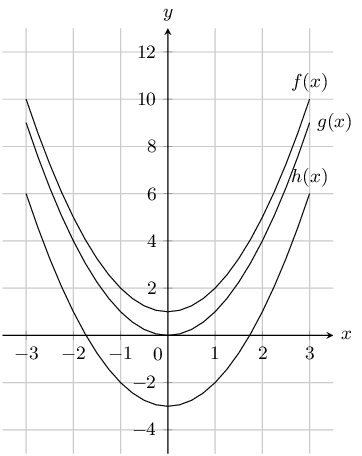$$y = x^2 - 3$$

$$h(x)$$

$$y = x^2 + 1$$

$$f(x)$$

$$y = x^2$$

$$g(x)$$

Two parabolas are drawn: $$g: y=ax^2+p$$ and $$h:y=bx^2+q$$.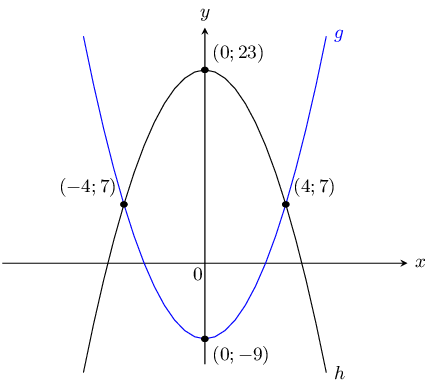Find the values of $$a$$ and $$p$$.

$$p$$ is the $$y$$-intercept of the function $$g(x)$$, therefore $$p=-9$$

To find $$a$$ we use one of the points on the graph (e.g. $$(4;7)$$):

\begin{align*} y&=ax^2-9\\ 7&= a(4^2) - 9\\ 16a&=16\\ \therefore a&=1 \end{align*}

$$a=1$$; $$p=-9$$

Find the values of $$b$$ and $$q$$.

$$q$$ is the $$y$$-intercept of the function $$h(x)$$, therefore $$q = 23$$

To find $$b$$, we use one of the points on the graph (e.g. $$(4;7)$$):

\begin{align*} y&=bx^{2} =23\\ 7&= b(4^{2}) +23\\ 16b&=-16\\ \therefore b&=-1 \end{align*}

$$b=-1$$; $$q=23$$

Find the values of $$x$$ for which $$g(x) \geq h(x)$$.

These are the points where $$g$$ lies above $$h$$.

From the graph we see that $$g$$ lies above $$h$$ when: $$x \le -4$$ or $$x \geq 4$$

For what values of $$x$$ is $$g$$ increasing?

$$g$$ increases from the turning point $$(0;-9)$$, i.e. for $$x \geq 0$$.

Show that if $$a < 0$$ the range of $$f(x)=ax^2 + q$$ is $$\left\{f(x):f(x) \le q\right\}$$.

Because the square of any number is always positive we get: $$x^2 \geq 0$$.

If we multiply by $$a$$ where $$(a < 0)$$ then the sign of the inequality is reversed: $$ax^2 \le 0$$

Adding $$q$$ to both sides gives $$ax^2 + q \le q$$

And so $$f(x) \le q$$

This gives the range as $$(-\infty; q]$$.

Draw the graph of the function $$y=-x^2 + 4$$ showing all intercepts with the axes.

The $$y$$-intercept is $$(0;4)$$. The $$x$$-intercepts are given by setting $$y = 0$$:

\begin{align*} 0 & = -x^2 + 4 \\ x^2 & = 4 \\ x & = \pm 2 \end{align*}

Therefore the $$x$$-intercepts are: $$(2;0)$$ and $$(-2;0)$$.

Now we can sketch the graph: